## Spherical mirrors; and Refraction

3-26-99

Sections 23.3 -23.5

### A ray diagram for a convex mirror

What happens with a convex mirror? In this case the ray diagram looks like this: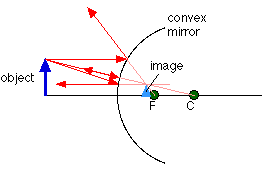As the ray diagram shows, the image for a convex mirror is virtual, and upright compared to the object. A convex mirror is the kind of mirror used for security in stores, and is also the kind of mirror used on the passenger side of many cars ("Objects in mirror are closer than they appear."). A convex mirror will reflect a set of parallel rays in all directions; conversely, it will also take light from all directions and reflect it in one direction, which is exactly how it's used in stores and cars.

### The mirror equation

Drawing a ray diagram is a great way to get a rough idea of how big the image of an object is, and where the image is located. We can also calculate these things precisely, using something known as the mirror equation. The textbook does a nice job of deriving this equation in section 25.6, using the geometry of similar triangles.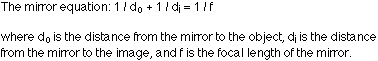### Magnification

In most cases the height of the image differs from the height of the object, meaning that the mirror has done some magnifying (or reducing). The magnification, m, is defined as the ratio of the image height to the object height, which is closely related to the ratio of the image distance to the object distance: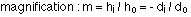A magnification of 1 (plus or minus) means that the image is the same size as the object. If m has a magnitude greater than 1 the image is larger than the object, and an m with a magnitude less than 1 means the image is smaller than the object. If the magnification is positive, the image is upright compared to the object; if m is negative, the image is inverted compared to the object.

### Sign conventions

What does a positive or negative image height or image distance mean? To figure out what the signs mean, take the side of the mirror where the object is to be the positive side. Any distances measured on that side are positive. Distances measured on the other side are negative.

f, the focal length, is positive for a concave mirror, and negative for a convex mirror.

When the image distance is positive, the image is on the same side of the mirror as the object, and it is real and inverted. When the image distance is negative, the image is behind the mirror, so the image is virtual and upright.

A negative m means that the image is inverted. Positive means an upright image.

### Steps for analyzing mirror problems

There are basically three steps to follow to analyze any mirror problem, which generally means determining where the image of an object is located, and determining what kind of image it is (real or virtual, upright or inverted).

• Step 1 - Draw a ray diagram. The more careful you are in constructing this, the better idea you'll have of where the image is.
• Step 2 - Apply the mirror equation to determine the image distance. (Or to find the object distance, or the focal length, depending on what is given.)
• Step 3 - Make sure steps 1 and 2 are consistent with each other.

### An example

A Star Wars action figure, 8.0 cm tall, is placed 23.0 cm in front of a concave mirror with a focal length of 10.0 cm. Where is the image? How tall is the image? What are the characteristics of the image?

The first step is to draw the ray diagram, which should tell you that the image is real, inverted, smaller than the object, and between the focal point and the center of curvature. The location of the image can be found from the mirror equation: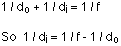which can be rearranged to: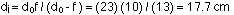The image distance is positive, meaning that it is on the same side of the mirror as the object. This agrees with the ray diagram. Note that we don't need to worry about converting distances to meters; just make sure everything has the same units, and whatever unit goes into the equation is what comes out.

Calculating the magnification gives: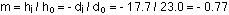Solving for the image height gives:The negative sign for the magnification, and the image height, tells us that the image is inverted compared to the object.

To summarize, the image is real, inverted, 6.2 cm high, and 17.7 cm in front of the mirror.

### Example 2 - a convex mirror

The same Star Wars action figure, 8.0 cm tall, is placed 6.0 cm in front of a convex mirror with a focal length of -12.0 cm. Where is the image in this case, and what are the image characteristics?

Again, the first step is to draw a ray diagram. This should tell you that the image is located behind the mirror; that it is an upright, virtual image; that it is a little smaller than the object; and that the image is between the mirror and the focal point.

The second step is to confirm all those observations. The mirror equation, rearranged as in the first example, gives: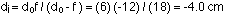Solving for the magnification gives: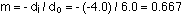This gives an image height of 0.667 x 8 = 5.3 cm.

All of these results are consistent with the conclusions drawn from the ray diagram. The image is 5.3 cm high, virtual, upright compared to the object, and 4.0 cm behind the mirror.

### Refraction

When we talk about the speed of light, we're usually talking about the speed of light in a vacuum, which is 3.00 x 108 m/s. When light travels through something else, such as glass, diamond, or plastic, it travels at a different speed. The speed of light in a given material is related to a quantity called the index of refraction, n, which is defined as the ratio of the speed of light in vacuum to the speed of light in the medium:

index of refraction : n = c / v

When light travels from one medium to another, the speed changes, as does the wavelength. The index of refraction can also be stated in terms of wavelength:Although the speed changes and wavelength changes, the frequency of the light will be constant. The frequency, wavelength, and speed are related by: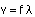The change in speed that occurs when light passes from one medium to another is responsible for the bending of light, or refraction, that takes place at an interface. If light is travelling from medium 1 into medium 2, and angles are measured from the normal to the interface, the angle of transmission of the light into the second medium is related to the angle of incidence by Snell's law :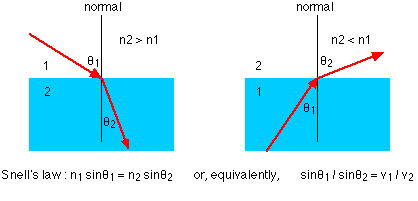When light crosses an interface into a medium with a higher index of refraction, the light bends towards the normal. Conversely, light traveling across an interface from higher n to lower n will bend away from the normal. This has an interesting implication: at some angle, known as the critical angle, light travelling from a medium with higher n to a medium with lower n will be refracted at 90°; in other words, refracted along the interface. If the light hits the interface at any angle larger than this critical angle, it will not pass through to the second medium at all. Instead, all of it will be reflected back into the first medium, a process known as total internal reflection.